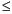Functions and CALL Routines

# RANUNI Function

Returns a random variate from a uniform distribution.
 Category: Random Number Tip: If you want to change the seed value during execution, you must use the CALL RANUNI routine instead of the RANUNI function.

## Syntax

 RANUNI(seed)

### Arguments

seed

is a numeric constant, variable, or expression with an integer value. If seed0, the time of day is used to initialize the seed stream.

The RANUNI function returns a number that is generated from the uniform distribution on the interval (0,1) using a prime modulus multiplicative generator with modulus 231- and multiplier 397204094 (Fishman and Moore 1982) (See References).

You can use a multiplier to change the length of the interval and an added constant to move the interval. For example,

`random_variate=a*ranuni(seed)+b; `

returns a number that is generated from the uniform distribution on the interval (b,a+b).

The CALL RANUNI routine, an alternative to the RANUNI function, gives greater control of the seed and random number streams.

Functions and CALL routines:Previous Page | Next Page | Top of Page